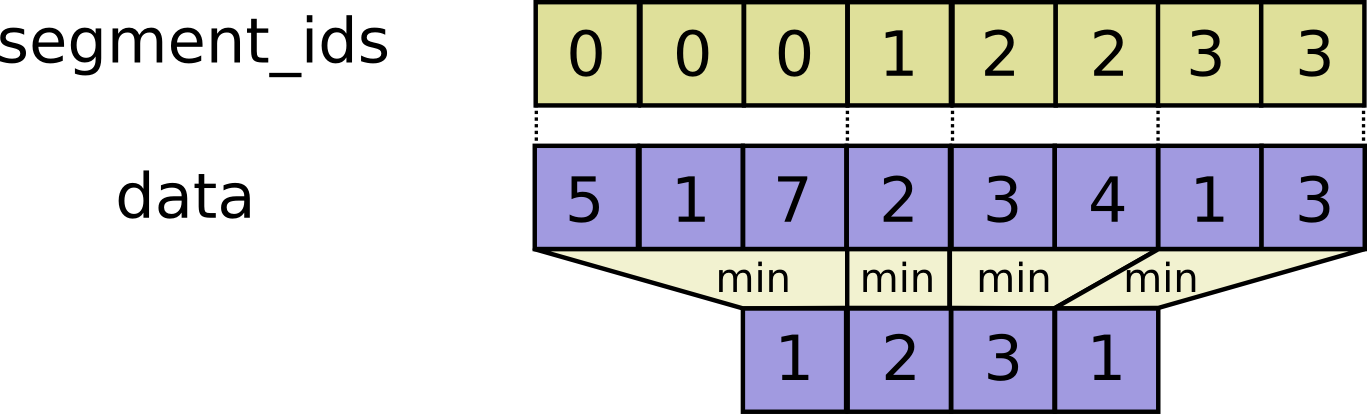# tf.math.segment_min

Computes the minimum along segments of a tensor.

Read the section on segmentation for an explanation of segments.

Computes a tensor such that $$output_i = \min_j(data_j)$$ where min is over j such that segment_ids[j] == i.

If the min is empty for a given segment ID i, output[i] = 0.#### For example:

c = tf.constant([[1,2,3,4], [4, 3, 2, 1], [5,6,7,8]])
tf.segment_min(c, tf.constant([0, 0, 1]))
# ==> [[1, 2, 2, 1],
#      [5, 6, 7, 8]]


data A Tensor. Must be one of the following types: float32, float64, int32, uint8, int16, int8, int64, bfloat16, uint16, half, uint32, uint64.
segment_ids A Tensor. Must be one of the following types: int32, int64. A 1-D tensor whose size is equal to the size of data's first dimension. Values should be sorted and can be repeated.
name A name for the operation (optional).

A Tensor. Has the same type as data.

[{ "type": "thumb-down", "id": "missingTheInformationINeed", "label":"Missing the information I need" },{ "type": "thumb-down", "id": "tooComplicatedTooManySteps", "label":"Too complicated / too many steps" },{ "type": "thumb-down", "id": "outOfDate", "label":"Out of date" },{ "type": "thumb-down", "id": "samplesCodeIssue", "label":"Samples / code issue" },{ "type": "thumb-down", "id": "otherDown", "label":"Other" }]
[{ "type": "thumb-up", "id": "easyToUnderstand", "label":"Easy to understand" },{ "type": "thumb-up", "id": "solvedMyProblem", "label":"Solved my problem" },{ "type": "thumb-up", "id": "otherUp", "label":"Other" }]